# 学习笔记CB009:人工神经网络模型、手写数字识别、多层卷积网络、词向量、word2vec

+关注继续查看

# coding:utf-8

import sys
import importlib

from tensorflow.examples.tutorials.mnist import input_data
import tensorflow as tf

flags = tf.app.flags
FLAGS = flags.FLAGS
flags.DEFINE_string('data_dir', './', 'Directory for storing data')

# 初始化生成随机的权重(变量)，避免神经元输出恒为0
def weight_variable(shape):
# 以正态分布生成随机值
initial = tf.truncated_normal(shape, stddev=0.1)
return tf.Variable(initial)

# 初始化生成随机的偏置项(常量)，避免神经元输出恒为0
def bias_variable(shape):
initial = tf.constant(0.1, shape=shape)
return tf.Variable(initial)

# 卷积采用1步长，0边距，保证输入输出大小相同
def conv2d(x, W):
return tf.nn.conv2d(x, W, strides=[1, 1, 1, 1], padding='SAME')

# 池化采用2×2模板
def max_pool_2x2(x):
return tf.nn.max_pool(x, ksize=[1, 2, 2, 1],

# 28*28=784
x = tf.placeholder(tf.float32, [None, 784])
# 输出类别共10个：0-9
y_ = tf.placeholder("float", [None,10])

# 第一层卷积权重，视野是5*5，输入通道1个，输出通道32个
W_conv1 = weight_variable([5, 5, 1, 32])
# 第一层卷积偏置项有32个
b_conv1 = bias_variable()

# 把x变成4d向量，第二维和第三维是图像尺寸，第四维是颜色通道数1
x_image = tf.reshape(x, [-1,28,28,1])

h_conv1 = tf.nn.relu(conv2d(x_image, W_conv1) + b_conv1)
h_pool1 = max_pool_2x2(h_conv1)

# 第二层卷积权重，视野是5*5，输入通道32个，输出通道64个
W_conv2 = weight_variable([5, 5, 32, 64])
# 第二层卷积偏置项有64个
b_conv2 = bias_variable()

h_conv2 = tf.nn.relu(conv2d(h_pool1, W_conv2) + b_conv2)
h_pool2 = max_pool_2x2(h_conv2)

# 第二层池化后尺寸编程7*7，第三层是全连接，输入是64个通道，输出是1024个神经元
W_fc1 = weight_variable([7 * 7 * 64, 1024])
# 第三层全连接偏置项有1024个
b_fc1 = bias_variable()

h_pool2_flat = tf.reshape(h_pool2, [-1, 7*7*64])
h_fc1 = tf.nn.relu(tf.matmul(h_pool2_flat, W_fc1) + b_fc1)

# 按float做dropout，以减少过拟合
keep_prob = tf.placeholder("float")
h_fc1_drop = tf.nn.dropout(h_fc1, keep_prob)

# 最后的softmax层生成10种分类
W_fc2 = weight_variable([1024, 10])
b_fc2 = bias_variable()

y_conv=tf.nn.softmax(tf.matmul(h_fc1_drop, W_fc2) + b_fc2)

cross_entropy = -tf.reduce_sum(y_*tf.log(y_conv))
correct_prediction = tf.equal(tf.argmax(y_conv,1), tf.argmax(y_,1))
accuracy = tf.reduce_mean(tf.cast(correct_prediction, "float"))

sess = tf.InteractiveSession()
sess.run(tf.global_variables_initializer())

for i in range(20000):
batch = mnist.train.next_batch(50)
if i%100 == 0:
train_accuracy = accuracy.eval(feed_dict={
x:batch, y_: batch, keep_prob: 1.0})
print("step %d, training accuracy %g"%(i, train_accuracy))
train_step.run(feed_dict={x: batch, y_: batch, keep_prob: 0.5})

print("test accuracy %g"%accuracy.eval(feed_dict={
x: mnist.test.images, y_: mnist.test.labels, keep_prob: 1.0}))


word embedding，词嵌入，范畴论，morphism(态射)，态射表示两个数学结构中保持结构过程抽象，一个域和另一个域之间关系。范畴论中嵌入(态射)保持结构，word embedding表示“降维”嵌入，通过降维避免维度灾难，降低计算复杂度，更易于深度学习应用。

word2vec本质，通过distributed representation表达方式表示词，通过降维word embedding减少计算量。

word2vec训练神经概率语言模型。word2vec CBOW和Skip-gram模型。CBOW模型。Continuous Bag-of-Words Model，已知当前词上下文预测当前词。CBOW模型神经网络结构，输入层，词w上下文2c个词的词向量。投影层，输入层2c个向量做求和累加。输出层，霍夫曼树，叶子节点是语料出现过词，权重是出现次数。神经网络模型首尾相接改成求和累加，减少维度。去掉隐藏层，减少计算量。输出层softmax归一化运算改成霍夫曼树。

word2vec，下载源码，https://github.com/warmheartli/ChatBotCourse/tree/master/word2vec)，执行make编译(mac系统代码所有#include 替换成#include )。编译生成word2vec、word2phrase、word-analogy、distance、compute-accuracy二进制文件。训练，语料，已切好词(空格分隔)文本。执行 ./word2vec -train train.txt -output vectors.bin -cbow 0 -size 200 -window 5 -negative 0 -hs 1 -sample 1e-3 -thread 12 -binary 1 。生成vectors.bin文件，训练好词向量二进制文件，求近义词了，执行 ./distance vectors.bin 。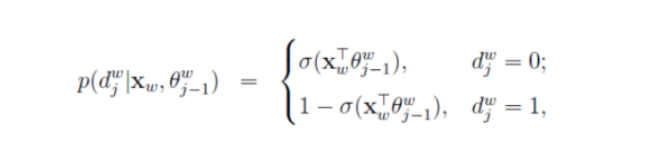word2vec模型原理及实现词向量训练案例（一）
word2vec模型原理及实现词向量训练案例
44 0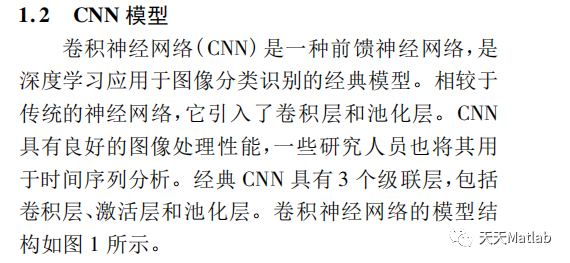【LSTM预测】基于卷积神经网络结合双向长短时记忆CNN-BiLSTM（多输入单输出）数据预测含Matlab源码
【LSTM预测】基于卷积神经网络结合双向长短时记忆CNN-BiLSTM（多输入单输出）数据预测含Matlab源码
69 0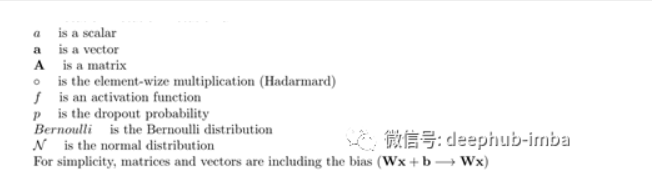DNN、CNN和RNN的12种主要dropout方法的数学和视觉解释（一）
DNN、CNN和RNN的12种主要dropout方法的数学和视觉解释（一）
29 0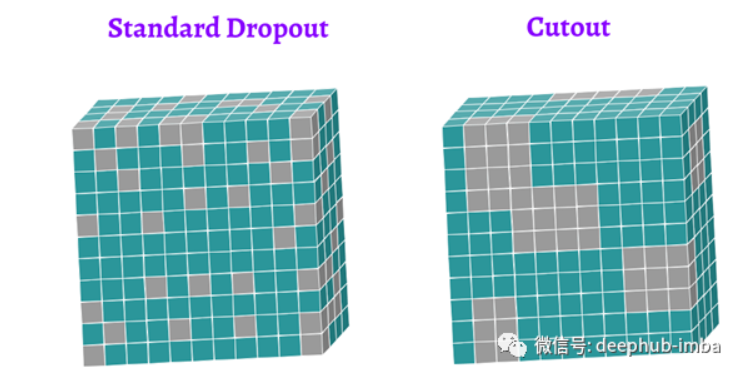DNN、CNN和RNN的12种主要dropout方法的数学和视觉解释（三）
DNN、CNN和RNN的12种主要dropout方法的数学和视觉解释（三）
70 0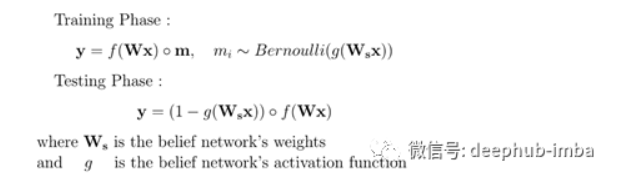DNN、CNN和RNN的12种主要dropout方法的数学和视觉解释（二）
DNN、CNN和RNN的12种主要dropout方法的数学和视觉解释（二）
62 0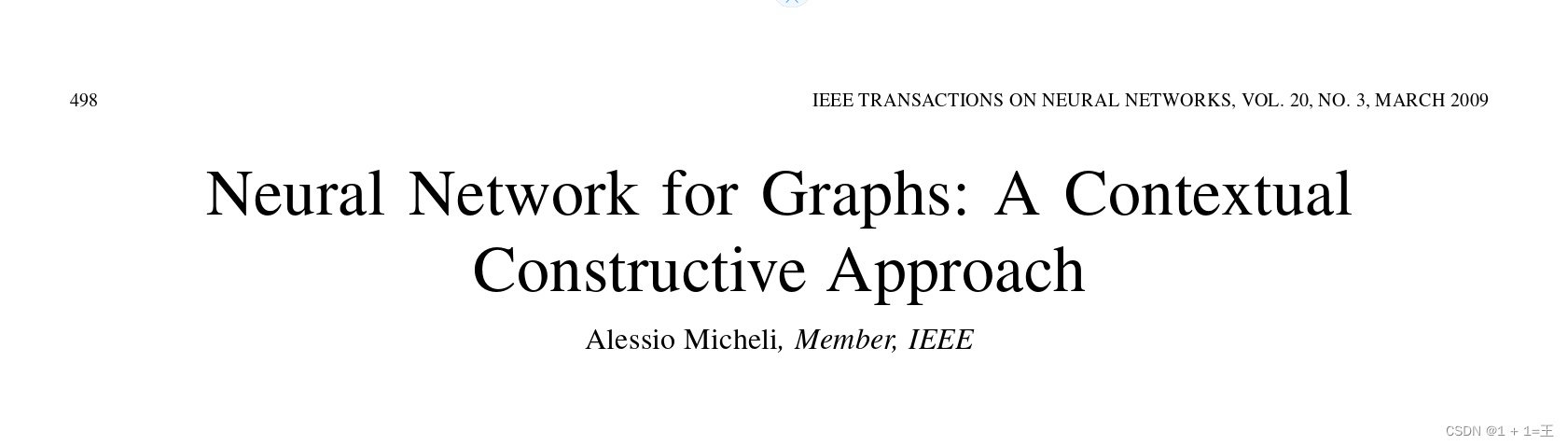【图神经网络】 - GNN的几个模型及论文解析（NN4G、GAT、GCN）
【图神经网络】 - GNN的几个模型及论文解析（NN4G、GAT、GCN）
120 0PGL图学习之图神经网络GNN模型GCN、GAT[系列六]

117 0DL之LSTM：基于《wonderland爱丽丝梦游仙境记》小说数据集利用LSTM算法(基于keras)对word实现预测
DL之LSTM：基于《wonderland爱丽丝梦游仙境记》小说数据集利用LSTM算法(基于keras)对word实现预测
78 0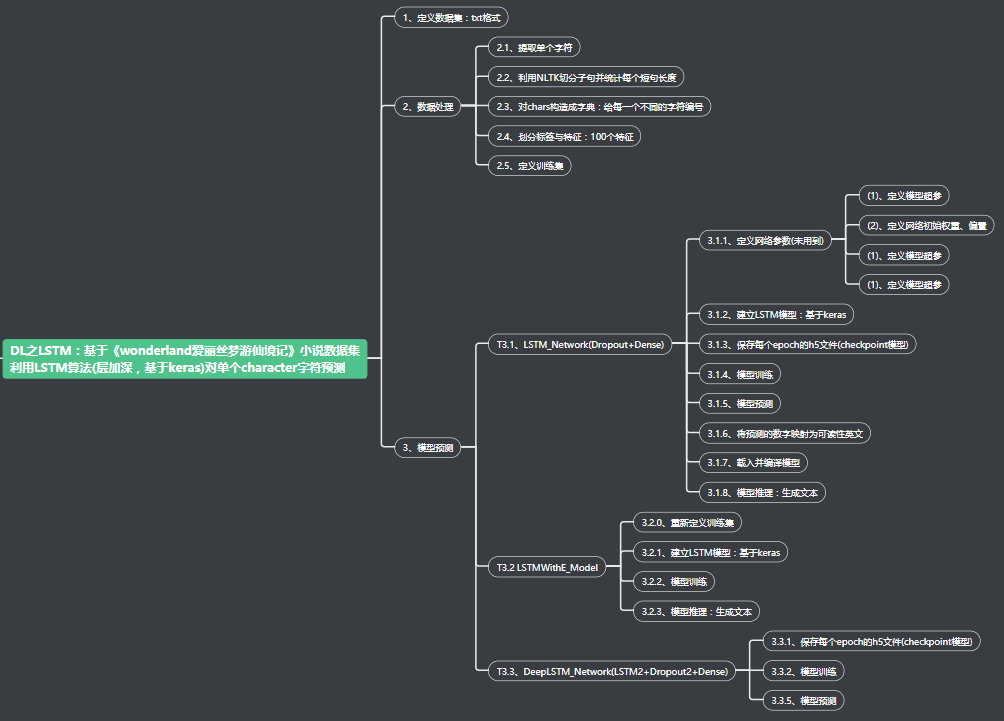DL之LSTM：基于《wonderland爱丽丝梦游仙境记》小说数据集利用LSTM算法(层加深，基于keras)对单个character字符预测
DL之LSTM：基于《wonderland爱丽丝梦游仙境记》小说数据集利用LSTM算法(层加深，基于keras)对单个character字符预测
69 05763675627

【算法实战】6. 支持向量机算法5410

【算法实战】9. 线性回归算法315

【算法实战】5. Logistic回归算法396

【算法实战】2. K近邻算法1325